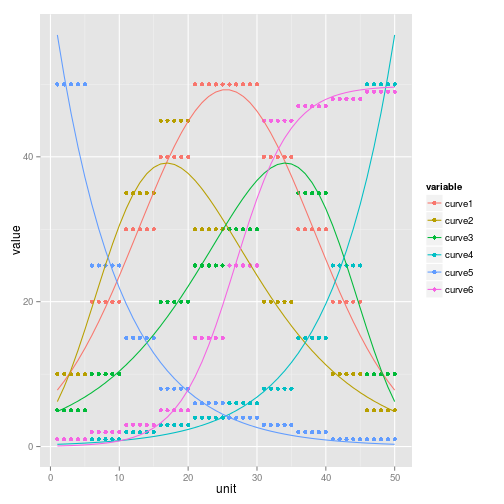# Curve fitting: R vs SPSS

Finding an equation or model to fit empirical data has been an ongoing concern of thinkers for centuries. Organised religion can be seen as example of this - God can provide a coherent explanation for droughts, dreams or death. With the development of natural science more rigorous, precise and testable hypothese about how the world works have been developed. Unlike vague verbal theology, scientific theories should to some extent be falsifiable. That massive bodies attract each other with a force equal to $$frac{m_1m_2}{r^2}$$, where m is the mass of two bodies and r is the distance between them, can be tested: if measurements based on this theory produce data that diverge from what the theory says in repeatable experiments, the theory has been proved to not always hold.

Such is the attraction of quantification and mathematical models in science: they force clearly stated and usually testable hypotheses that can be seen as rigorous. In the social sciences falsifiability is rare but something which many academics strive towards. It very course terms if mathematics is the language of nature, as some physicists would argue, then quantitative measurement and testing can make our research more closely bound to reality. As Leonardo da Vinci put it: “There is no certainty where one can neither apply any of the mathematical sciences nor any of those that are based on the mathematical sciences” Manuscript G, quoted in (Rosci, 1978, p. 13).

This context helps explain the continued importance and popularity of fitting mathematical models to data in many branches of science. There are dangers associated with unthinking application of this method, however: the term “curve fitting” can be used disparagingly, to criticise research that seeks to fit an equation, any equation, to a given dataset without any deep consideration of the underlying processes or complexity of the phenomena being observed. “With four parameters I can fit an elephant, and with five I can make him wiggle his trunk”, as von Neumann famously said.

Still, curve fitting is extremely useful in many contexts if process leads to greater understanding, testable hypotheses and reproducibility of research. It is worth trying to put our ideas into equations and attempting to fit equations to the observations can help with this process. “If a picture is worth a thousand words (as well as millions of pixels) then - as is shown later - an equation can be worth a billion pictures” (Flake, 1998). So, based on some somewhat arbitrary datasets that looks like it contains some kind of underlying pattern provided by Paul Norman, let us forge forward and try to find mathematical expressions that capture their ebs and flows. We will see how to do this in R first, and then compare how this is done in another piece of statistical software: SPSS.

## Read in and visualise the data

Having a basic understanding of the form of the data is vital to subsequently modelling so getting this stage right is vital. For reproducibility, every single command run in R is shown, including the loading of packages not provided in R's base installation:

# library(foreign) # the most common way of loading spss files in R -
library(memisc)  # an alternative package to import spss files

## Loading required package: lattice
##
## Attaching package: 'memisc'
##
## The following objects are masked from 'package:stats':
##
##     contrasts, contr.sum, contr.treatment
##
## The following object is masked from 'package:base':
##
##     as.array

curves <- as.data.frame(as.data.set(spss.system.file("Curve-examples-Table1.sav")))
head(curves)  # view 1st 6 rows of data to check it makes sense

##   unit curve1 curve2 curve3 curve4 curve5 curve6
## 1    1     10     10      5      1     50      1
## 2    2     10     10      5      1     50      1
## 3    3     10     10      5      1     50      1
## 4    4     10     10      5      1     50      1
## 5    5     10     10      5      1     50      1
## 6    6     20     25     10      1     25      2

attach(curves)  # make column names available to the global environment
plot(unit, curve1)  # preliminary visualisation of 1st curve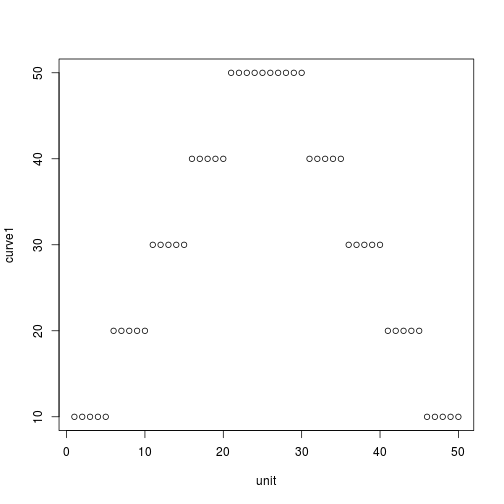library(reshape2)  # package for data manipulation
minput <- melt(curves, id.vars = "unit")  # put datafram into 'long form' with unit as id variable
# now plot the data: first all in one graph, then in 6 facetted graphs for
# clarity
library(ggplot2)  # Hadley Wickham's implementation of the 'grammar of graphics'
qplot(x = unit, y = value, color = variable, geom = "line", data = minput)  # bit of a mess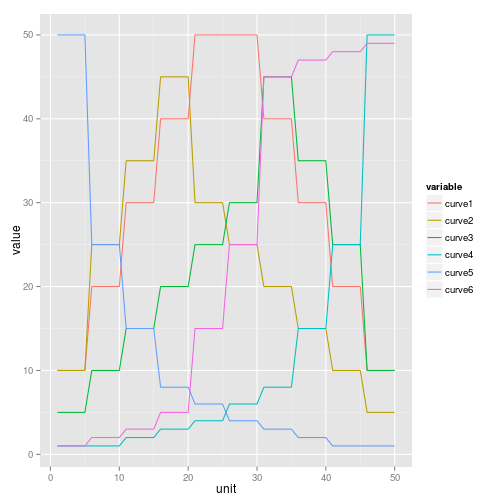ggplot(aes(x = unit, y = value), data = minput) + geom_line() + facet_grid(. ~
variable)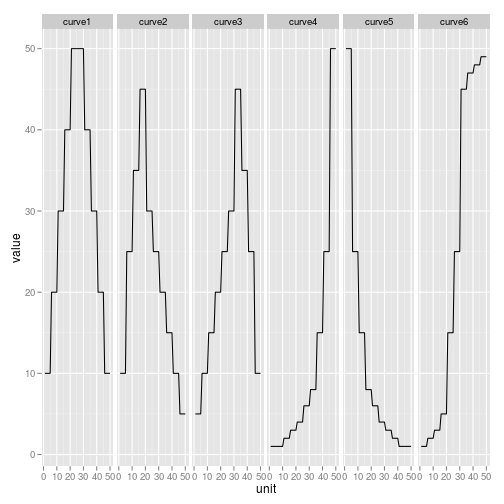Now we have the measure of the data, 50 observations of 6 variables, we proceed to fit equations to them.

## Fitting equations to the data by altering their coefficients

m1 <- nls(curve1 ~ I(a * exp(-(unit - b)^2/c)), data = curves, start = list(a = 50,
b = 25, c = 0.5), trace = T)

## 51235 :  50.0 25.0  0.5
## 46674 :  49.900 25.000  2.116
## 41621 :  35.714 25.000  8.058
## 27372 :  36.60 25.04 38.66
## 8202 :   42.11  25.26 142.67
## 689.7 :   47.44  25.47 288.18
## 382.1 :   49.28  25.50 323.98
## 381.9 :   49.29  25.50 325.41
## 381.9 :   49.29  25.50 325.45
## 381.9 :   49.29  25.50 325.45

plot(unit, curve1)
lines(unit, predict(m1, unit))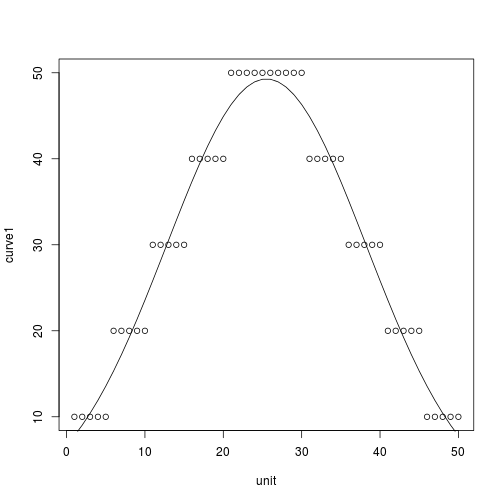# the number of input parameters of this graph were reduced to 3 it seemed
# to have too many degrees of freedom with 4
m2 <- nls(curve2 ~ I(a * exp(-b * (unit - c) - exp(-b * (unit - c)))), data = curves,
start = list(a = 50, b = 0.5, c = 20), trace = T)

## 25188 :  50.0  0.5 20.0
## 24546 :  80.9788 -0.8054 19.3669
## 23375 :  96.8777  0.7345 18.4871
## 16167 :  96.4162 -0.3469 18.6630
## 7909 :  102.8204   0.0411  17.6374
## 1079 :  98.21954  0.08102 18.93483
## 604.1 :  104.28814   0.08968  16.63686
## 585.8 :  106.28715   0.09169  16.89123
## 585.7 :  106.41593   0.09185  16.87624
## 585.7 :  106.42231   0.09186  16.87609
## 585.7 :  106.42275   0.09187  16.87607

plot(unit, curve2)
lines(unit, predict(m2, unit))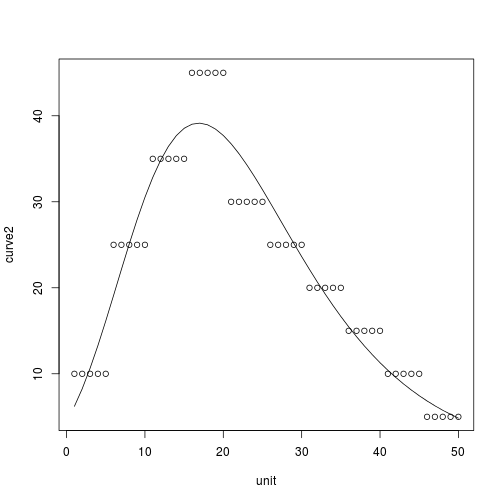# again, d.f. reduced to 3
m3 <- nls(curve3 ~ I(h * exp(-ra * (unit - p) - exp(-ra * (unit - p)))), start = list(h = 50,
ra = 0.5, p = 30), trace = T)

## 25014 :  50.0  0.5 30.0
## 21835 :  56.9376 -0.3585 31.5485
## 16990 :  90.3177  0.3556 32.3000
## 949.1 :  102.44780  -0.07743  33.41291
## 591.4 :  105.2442  -0.0899  34.1574
## 585.8 :  106.33340  -0.09172  34.11907
## 585.7 :  106.41707  -0.09186  34.12374
## 585.7 :  106.42238  -0.09186  34.12392
## 585.7 :  106.42275  -0.09187  34.12393

plot(unit, curve3)
lines(unit, predict(m3, unit))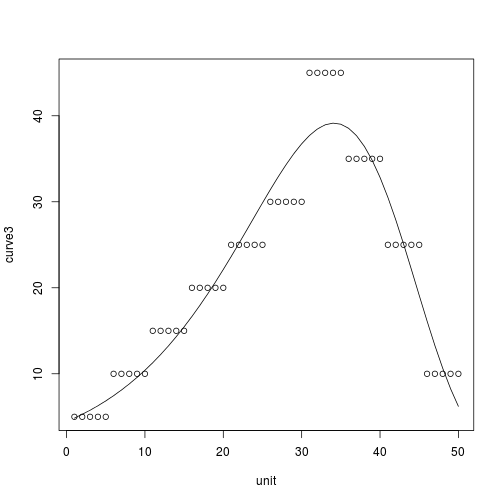# This curve seems to fit with only one coefficient to calculate
m4 <- nls(curve4 ~ I(exp(r * unit)), data = curves, start = list(r = 0.5), trace = T,
algorithm = "plinear")

## 8046 :  5.000e-01 1.068e-09
## 3305 :  0.2489063 0.0002927
## 1361 :  0.06591 1.65008
## 541.7 :  0.09481 0.47137
## 491.6 :  0.1050 0.2965
## 491.3 :  0.1059 0.2848
## 491.3 :  0.1059 0.2845
## 491.3 :  0.1059 0.2845

plot(unit, curve4)
lines(unit, predict(m4, unit))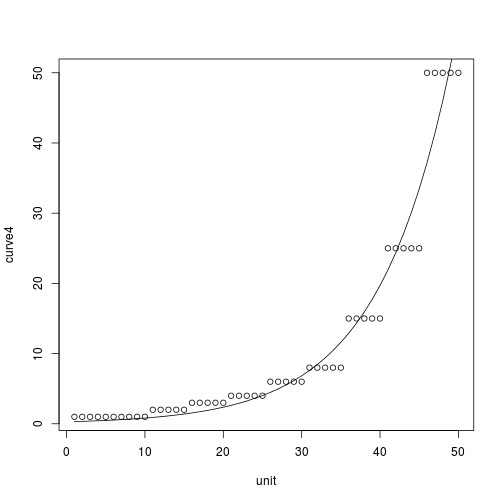m5 <- nls(curve5 ~ I(h * exp(-r * unit)), start = list(h = 50, r = 0.5), trace = T)

## 11480 :  50.0  0.5
## 8100 :  39.7217  0.2487
## 3218 :  40.4490  0.1187
## 1094 :  51.7129  0.1076
## 491.4 :  63.1870  0.1056
## 491.3 :  63.1788  0.1059
## 491.3 :  63.1814  0.1059

plot(unit, curve5)
lines(unit, predict(m5, unit))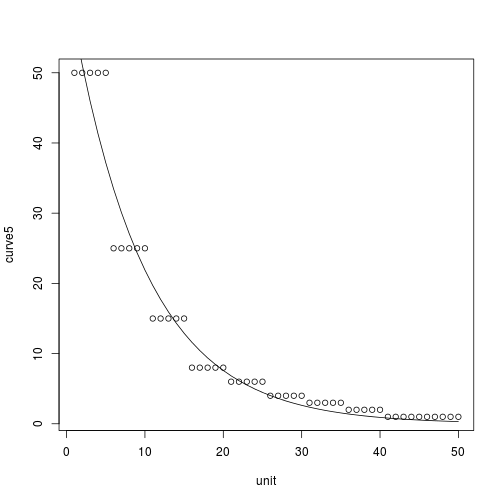# This worked fine, after a different algorithm was used to iterate through
# the input values
m6 <- nls(curve6 ~ I(a/(1 + exp(b + d * unit))), start = list(a = 50, b = 0.5,
d = 0.5), trace = T, algorithm = "port")

##   0:     24688.879:  50.0000 0.500000 0.500000
##   1:     24602.314:  40.1910 0.772446 0.577138
##   2:     24567.526:  18.9501  1.27360 0.416862
##   3:     24565.508:  14.9612  1.31679 0.357863
##   4:     24548.149:  11.0957  1.34722 0.182677
##   5:     15272.212:  9.34706  1.37247 -0.282810
##   6:     8942.9170:  19.3424  1.42406 -0.109874
##   7:     3809.4752:  29.4984  6.46778 -0.363110
##   8:     2109.7715:  33.7051  7.48109 -0.339375
##   9:     490.89687:  45.7340  7.19260 -0.258185
##  10:     194.87258:  49.6132  6.68494 -0.250717
##  11:     194.09177:  49.8279  6.58213 -0.245411
##  12:     194.07298:  49.7938  6.60116 -0.246293
##  13:     194.07290:  49.7938  6.60309 -0.246367
##  14:     194.07290:  49.7943  6.60289 -0.246356
##  15:     194.07290:  49.7942  6.60293 -0.246358
##  16:     194.07290:  49.7942  6.60293 -0.246358

plot(unit, curve6)
lines(unit, predict(m6, unit))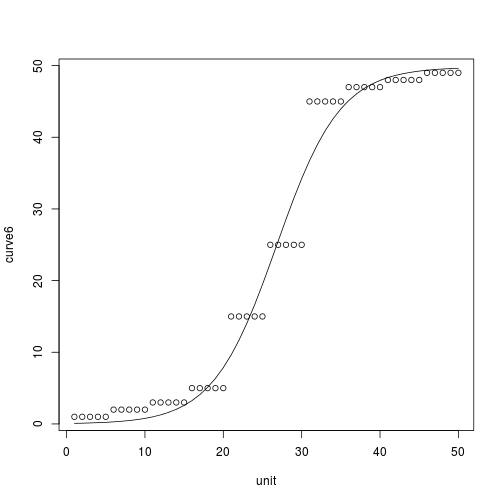This shows that all the synthetic data could be modelled. The data are unrealistically simple: real data is much messier, raising of which functional form to fit to the data and, more fundamentally, whether curve fitting with above a few degrees of freedom is worth it all.

In any case, such excersises can improve our understanding of how mathematical formula can relate to real world data, showcase available software for modelling and produce some attractive curves, as show below:

minput\$predicted <- c(predict(m1, unit), predict(m2, unit), predict(m3, unit),
predict(m4, unit), predict(m5, unit), predict(m6, unit))
ggplot(aes(x = unit), data = minput) + geom_point(aes(y = value)) + geom_line(aes(y = predicted)) +
facet_grid(. ~ variable)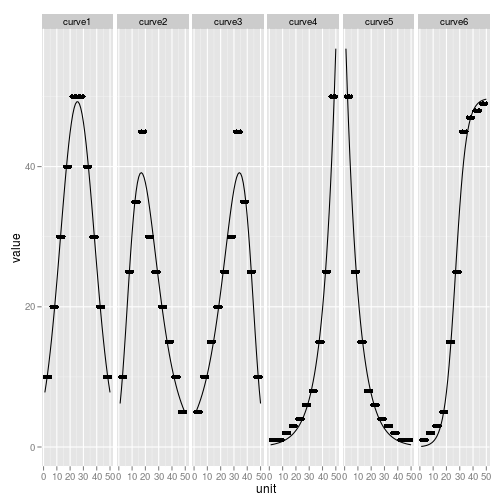# Now all together!
ggplot(aes(x = unit, color = variable), data = minput) + geom_point(aes(y = value)) +
geom_line(aes(y = predicted))